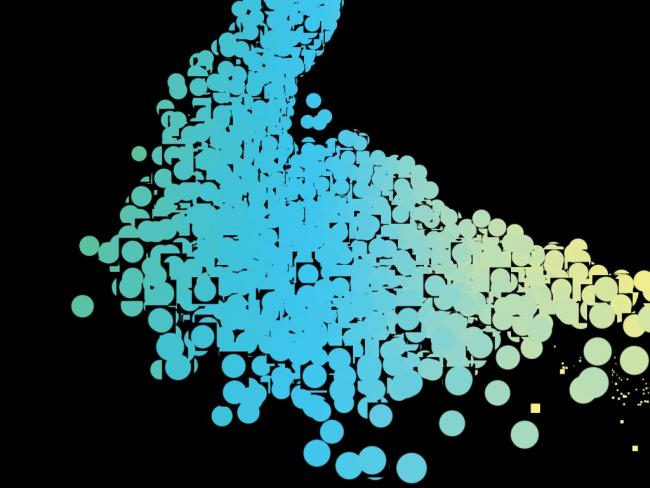#### Howdy, Stranger!

We are about to switch to a new forum software. Until then we have removed the registration on this forum.

# how to avoid transparent pixels to appear black

edited August 2015

Hello,

I'm using shaders to display rectangles as circles using a vertex point shader (frag shader) but depending of the angle of my camera the transparent zones appear as black (see below). Is there a way to fix this (using a shader) ?Tagged:

• I believe this problem occurs when rendering non z-sorted semi-transparent objects. You could try disabling the depth buffer inside your setup() function:

`hint(DISABLE_DEPTH_MASK);`

I think I saw this trick in one of the example sketch that comes with Processing.

• edited August 2015

This solution can work in some cases but I need to let it enabled. I was wondering if I can resolve this problem inside the shader without losing the depth information and let appear some particles on top of others.

• There should be multiple ways to fix that problem... depending on how your shader code looks like. Could you provide some example code for us to play around with? :)

• Here is a little example sketch using a modified version of the point shader found in Processing's Shader Tutorial:

``````class Particle {
float posX, posY, posZ;
float noiseOff = random(10000);
Particle(float posX, float posY, float posZ) {
this.posX = posX; this.posY = posY; this.posZ = posZ;
}
void draw() {
noiseOff += 0.01; posX += noise(noiseOff); posY += noise(noiseOff + 100.0); posZ += noise(noiseOff - 100.0);
posX = posX < -300.0 ? 300.0 : posX > 300.0 ? -300.0 : posX;
posY = posY < -300.0 ? 300.0 : posY > 300.0 ? -300.0 : posY;
posZ = posZ < -300.0 ? 300.0 : posZ > 300.0 ? -300.0 : posZ;
point(posX, posY, posZ);
}
}

ArrayList<Particle> particles = new ArrayList<Particle>();
float camAngle = 0.0;

void setup() {
size(800, 800, P3D);
"uniform mat4 projection;",
"uniform mat4 modelview;",
"uniform float weight;",
"attribute vec4 vertex;",
"attribute vec4 color;",
"attribute vec2 offset;",
"varying vec4 vertColor;",
"varying vec2 texCoord;",
"void main() {",
"vec4 pos = modelview * vertex;",
"vec4 clip = projection * pos;",
"gl_Position = clip + projection * vec4(offset, 0, 0);",
"texCoord = (vec2(0.5) + offset / weight);",
"vertColor = color;",
"}"
}, new String[] {
"#ifdef GL_ES",
"precision mediump float;",
"precision mediump int;",
"#endif",
"uniform vec2 screenInverse;",
"varying vec4 vertColor;",
"varying vec2 texCoord;",
"void main() {",
"gl_FragColor = vec4(gl_FragCoord.x * screenInverse.x, gl_FragCoord.y * screenInverse.y, 1.0, 1.0);",
"}"
});
for(int n = 1000; n-- > 0;)
particles.add(new Particle(random(-300.0, 300.0), random(-300.0, 300.0), random(-300.0, 300.0)));
strokeWeight(25);
stroke(255);
}

void draw() {
background(0);
camAngle += 0.004;
pointShader.set("screenInverse", 1.0 / width, 1.0 / height);
camera(sin(camAngle) * 1000.0, sin(camAngle * 0.2) * 500.0, cos(camAngle) * 1000.0, 0.0, 0.0, 0.0, 0.0, 1.0, 0.0);